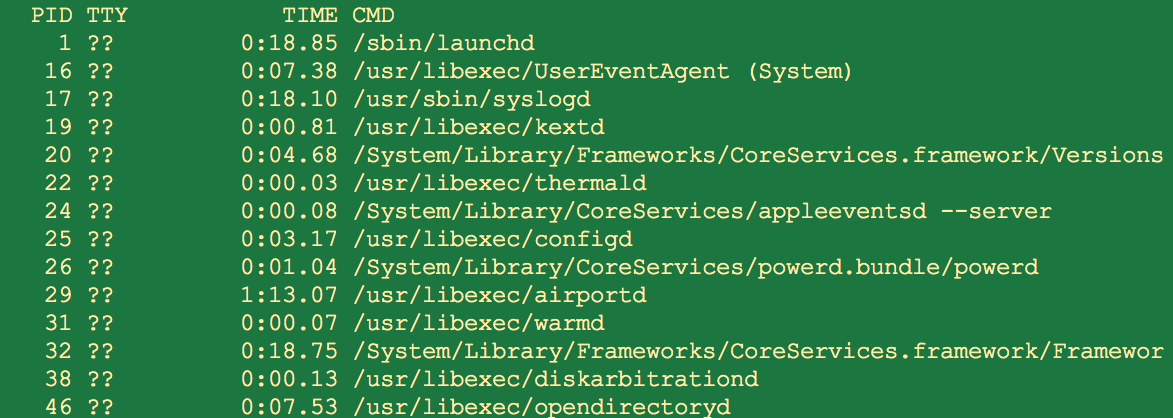# Getpid In Python

os.getpid

os.getpid()

None

• ## Process Id or pid is consumed by several methods like os.kill(), os.getsid() and so on.

• The following screenshot lists all the processes running under the operating system ( the list that can be shown to the current user)  along with the process Ids as given by the command ps -A.## Example 1:

 import os   print("PID of the current process is:") print(os.getpid())

## Output:

 PID of the current process is: 2842

## Example 2:

 import os   retVal  = os.fork() pid     = os.getpid()   if retVal is 0:     print("PID of the child process is: %d"%(pid)) else:     pid     = os.getpid()     print("PID of the parent process is: %d"%(pid))

## Output:

 PID of the parent process is: 2850 PID of the child process is: 2851